## 前言

“Actuaries always make assumptions before they solve questions. So I may assume that my girlfriend exists first.”

“I don't have girlfriend now，but maybe I will find one here during the period of master degree study.”1. 可以选择不表白。1. 如果表白呢？

## 1.Zero-sum two-player games 双方零和博弈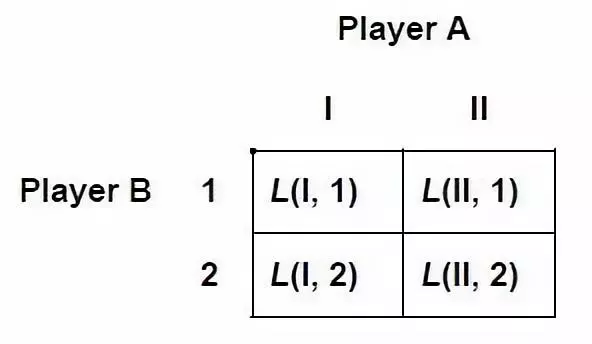### 1.1 Domination 占优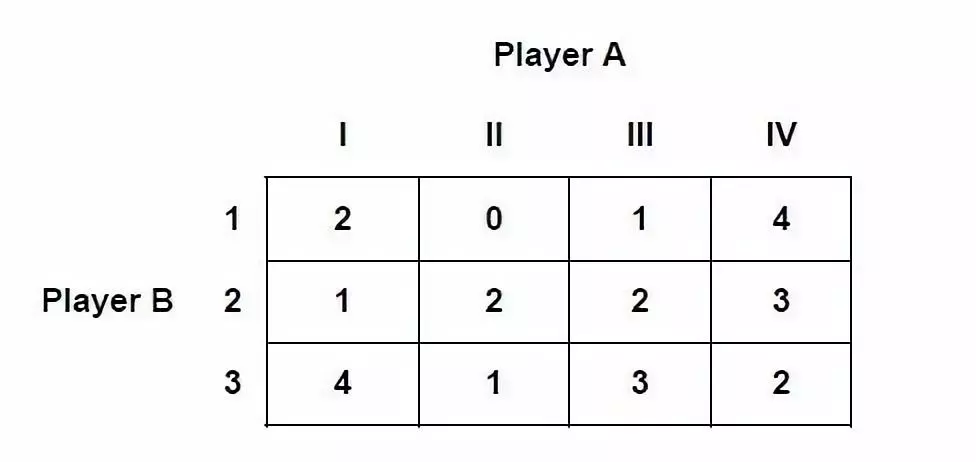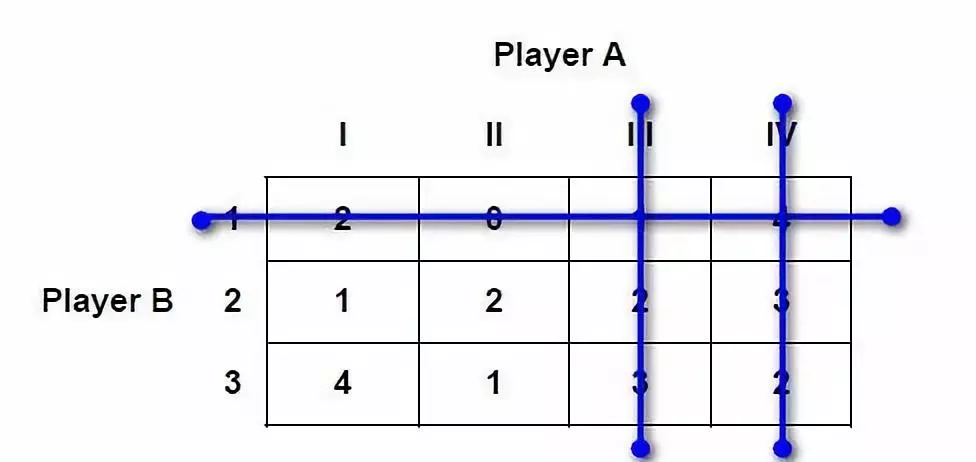### 1.2 The Minimax criterion 最小最大法则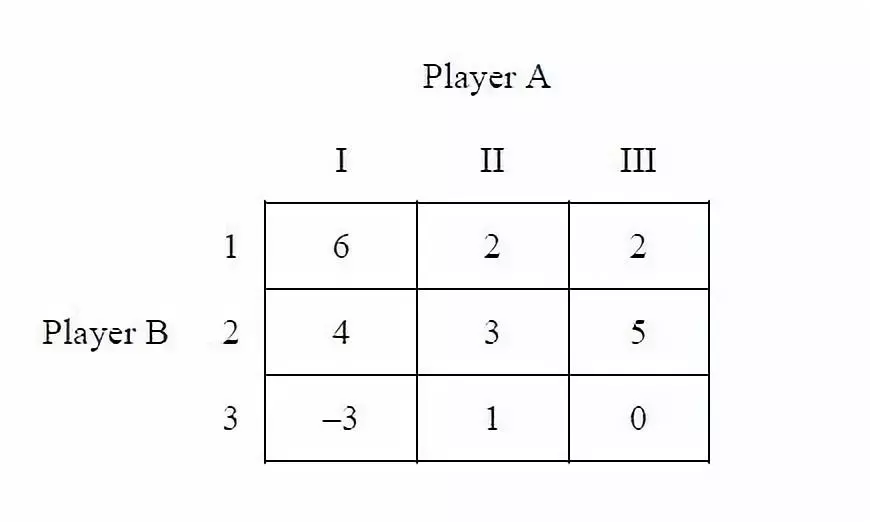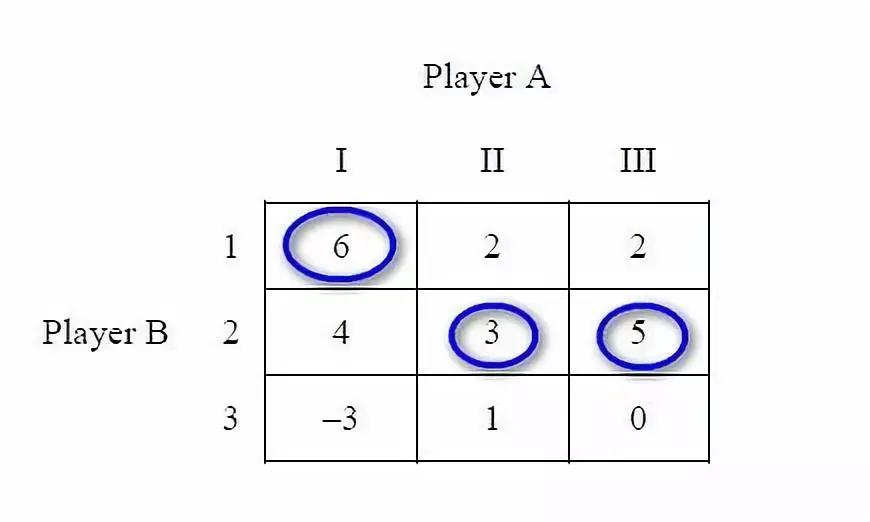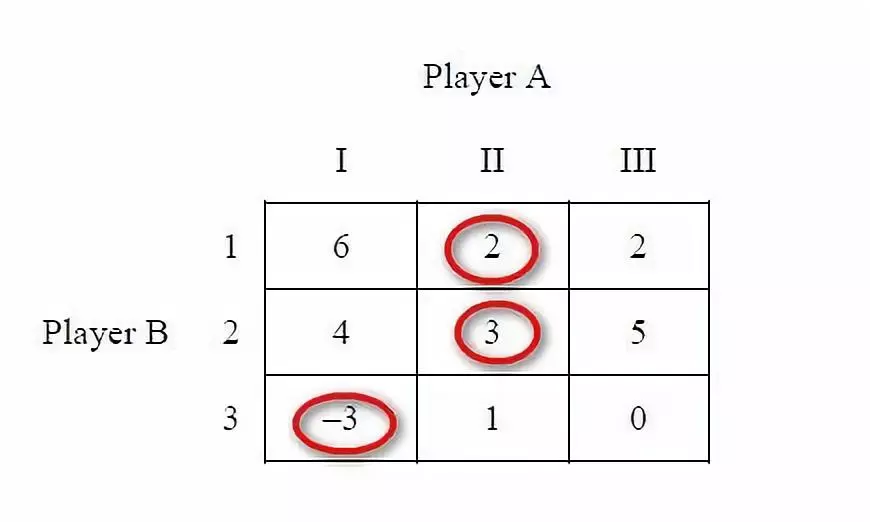### 1.3 Randomised Strategies 随机化策略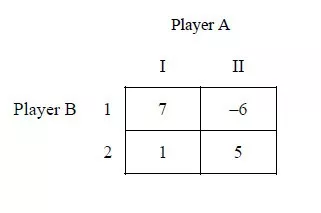## 2.Statistical games 统计博弈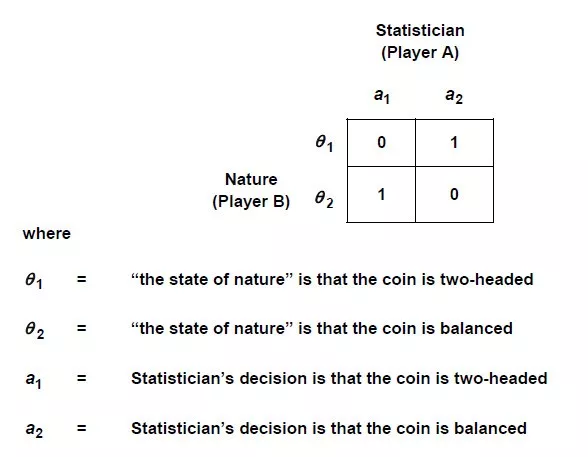$d_{1}(x)=\left\{\begin{array}{l} a_{1} \text { when } x=0 \\ a_{2} \text { when } x=1 \end{array}\right.$

- d2(0) =a1 and d2(1) =a1； - d3(0) =a2 and d3(1) =a2； - d4(0) =a2 and d4(1) =a1。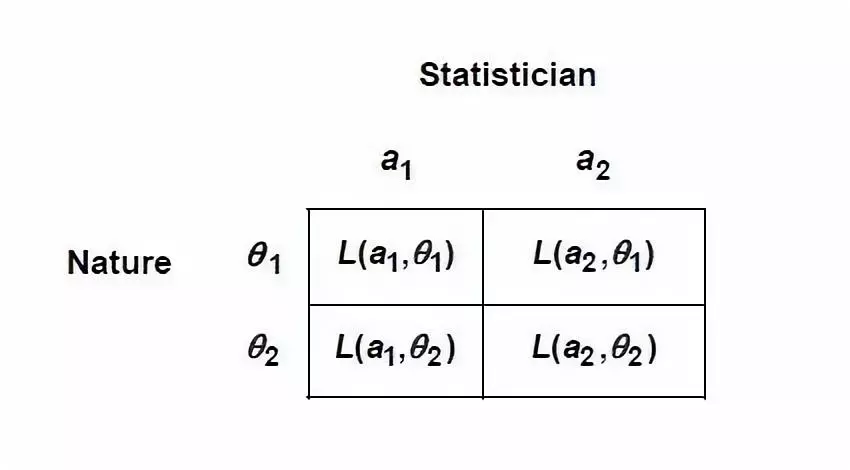$R\left(d_{1}, \theta_{j}\right)=E\left[L\left(d_{1}(x), \theta_{j}\right)\right]$

R称为风险函数Risk function，为期望损失。要计算出这些损失的期望，需要知道各损失函数的概率。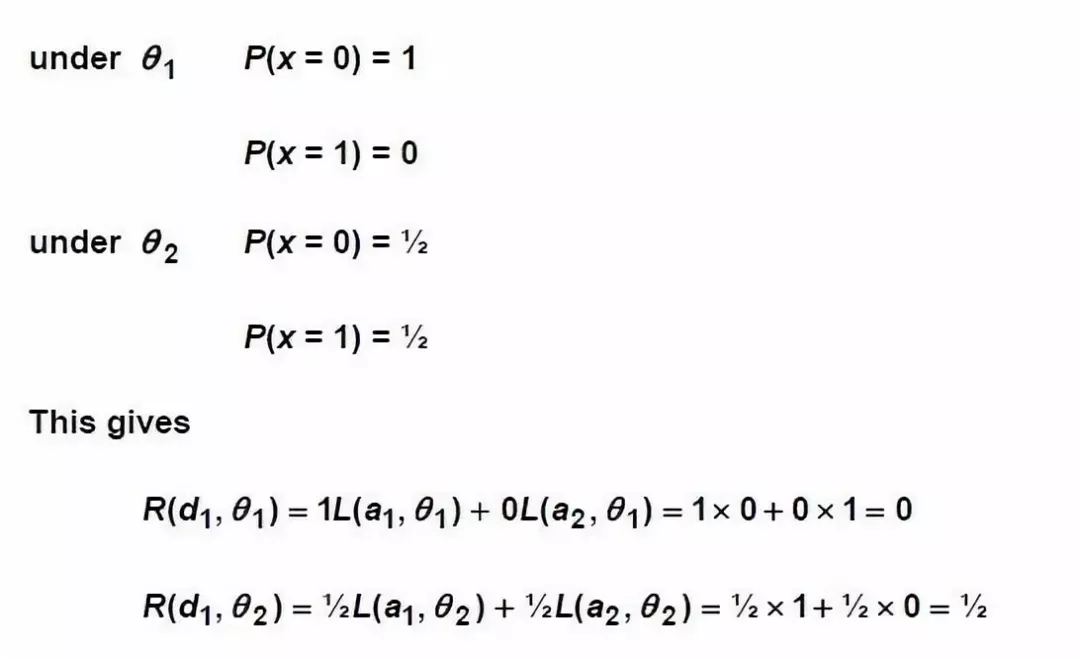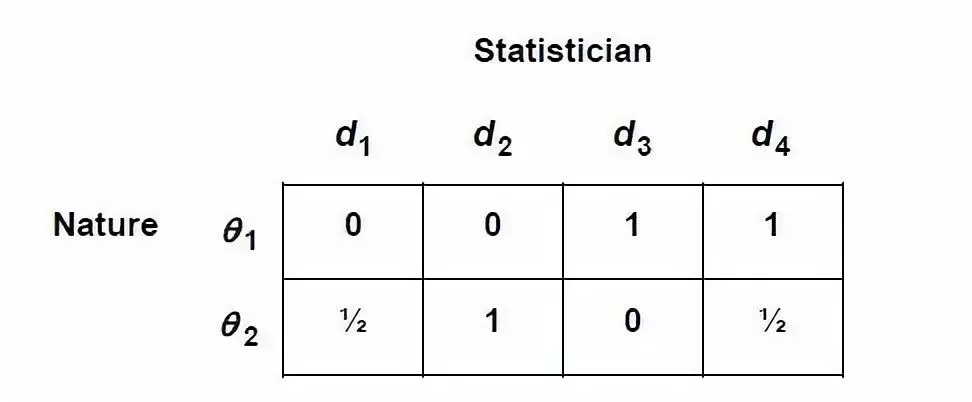• d1 dominates d2；
• d3 dominates d4。 此时鞍点不存在，所以我们可以用随机化策略确定最优策略：采取决策函数d1和d3的概率分别为2/3和1/3（用上文的方法自己算一下吧）。

## 3.Decision criteria 决策法则

### 3.1 The minimax criterion 最小最大法则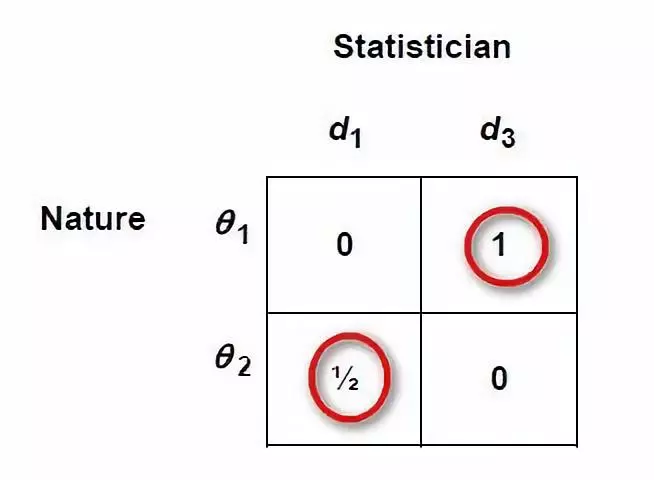### 3.2 The Bayes criterion 贝叶斯法则